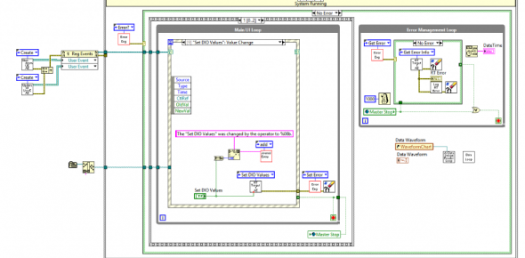# Common Tools In LabVIEW: Trivia Questions Quiz

30 Questions | Total Attempts: 442SettingsLabVIEW is a programming environment that the programmer the opportunity to create graphical diagrams and not texts like it is with the C and C++ languages. The trivia quiz below tests a learner’s ability to identify some of the common tools in LabVIEW. If you feel like you are up to the challenge, all you have to do is press start and choose the best answer to a question.

• 1.
To change the representation type of a number, right-click the control, indicator, or constant, and select ______.
• A.

Data operations

• B.

Representation

• C.

Numeric Pallete

• D.

Change Representation

• 2.
Floating-point numbers represent fractional numbers. In LabVIEW, floating-point numbers are represented with the color ___.
• A.

Orange

• B.

Red

• C.

Blue

• D.

Green

• 3.
When you wire two or more numeric inputs of different representations to a function, the function usually returns the data in the smaller or narrower representation.
• A.

True

• B.

False

• 4.
What floating-point numbers will you use when memory savings are important and so you will not overflow the range of the numbers?
• A.

EXT

• B.

EPL

• C.

DBL

• D.

SGL

• 5.
What is the default format for numeric objects?
• A.

Double-precision

• B.

Single-precision

• C.

Virtual precision

• D.

Extended precision

• 6.
It is a numeric data type that represents a set of rational numbers using binary digits, or bits.
• A.

Floating point

• B.

Dynamic integer

• C.

Fixed-point

• D.

Static integer

• 7.
In LabVIEW, the following are types of complex numbers except
• A.

CSL

• B.

CSG

• C.

CDB

• D.

CXT

• 8.
Quad unsigned integer numbers have ____ bits of storage.
• A.

16

• B.

32

• C.

64

• D.

128

• 9.
Long unsigned integer numbers have ____ bits of storage.
• A.

16

• B.

32

• C.

64

• D.

128

• 10.
Use unsigned integer data types when you know the integer is
• A.

Always positive

• B.

Always negative

• C.

Either positive or negative

• D.

An absolute value

• 11.
LabVIEW accepts a maximum word length of _____ bits.
• A.

32

• B.

64

• C.

128

• D.

256

• 12.
In LabVIEW, integers are represented with the color ____.
• A.

Black

• B.

Blue

• C.

Orange

• D.

Green

• 13.
What color represents Boolean data in LabVIEW?
• A.

Green

• B.

Orange

• C.

Blue

• D.

Red

• 14.
Which mechanical action changes the Boolean control value each time you click it with the Operating tool?
• A.

Switch when pressed

• B.

Switch when released

• C.

Latch when pressed

• D.

Latch when released

• 15.
Which mechanical action is similar to a circuit breaker and is useful for stopping a While Loop or for getting the VI to perform an action only once each time you set the control?
• A.

Switch when pressed

• B.

Switch when released

• C.

Latch when pressed

• D.

Latch when released

• 16.
Which mechanical action changes the Boolean control value when you click it and retains the new value until you release the mouse button?
• A.

Switch when pressed

• B.

Switch when released

• C.

Switch until released

• D.

Latch until released

• 17.
What is a sequence of displayable  or non-displayable ASCII characters that provides a platform-independent format for information and data?
• A.

Text message

• B.

String

• C.

Floating-point data

• D.

Integer

• 18.
What LabVIEW data types are represented with the color pink?
• A.

Strings

• B.

Integers

• C.

Floating-point data

• D.

Boolean data

• 19.
What is an output terminal that contains the number of completed iterations?
• A.

Iteration terminal

• B.

Loop terminal

• C.

Sampled terminal

• D.

Tunnel

• 20.
The image shows an equivalent flowchart of ____
• A.

For loop

• B.

While loop

• C.

Do loop

• D.

Do-while loop

• 21.
An express VI that keeps track of time while the VI continues to execute.
• A.

Elapsed time

• B.

Time delay

• C.

Enum

• D.

Tact time

• 22.
What will you use when you want to pass values from previous iterations through the loop to the next iteration?
• A.

Shift registers

• B.

Loops

• C.

Enums

• D.

Time delay

• 23.
Another method for retaining information from a previous iteration is ____
• A.

Feedback nodes

• B.

Time delay

• C.

Enums

• D.

Shift registers

• 24.
What chart mode works similarly to a scope chart except it shows the old data on the right and the new data on the left separated by a vertical line?
• A.

Sweep chart

• B.

Strip chart

• C.

Scale chart

• D.

Scope chart

• 25.
Which chart mode shows running data continuously scrolling from left to right across the chart with old data on the left and new data on the right?
• A.

Strip chart

• B.

Sweep chart

• C.

Scale chart

• D.

Scope chart

Related TopicsBack to top